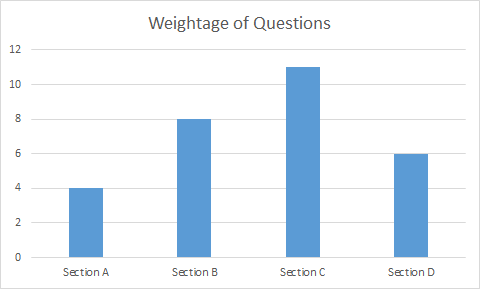# CBSE Class 12 Maths Post Paper Analysis 2019

The Central Board of Secondary Education (CBSE) conducted the Class 12th maths paper today i.e. Monday, 18th March 2019. The students from Class 12 Science as well as Commerce stream appeared for the examination at various centres across India. The exam was conducted from 10.30 am to 1.30 pm. For the CBSE Class 12 Science stream students the Maths paper marked the end of 2019 board examination.

As per the last year CBSE Class 12 Maths Question Paper Analysis 2018 the exam level was tough which left students confused.

## CBSE Class 12 Maths Paper Difficulty Level Analysis

CBSE Class 12 Maths paper was for a total of 100 marks. According to some students’ the Maths paper was a bit tricky and difficult. Some of the questions were very confusing while some are very easy. As per some students the Maths paper was very easy as they are expecting some tough questions in Section D. Some students found it a big lengthy and they are not able to complete it on time. As per the analysis, few of the questions need high level thinking to solve it. Few of the questions were picked up from CBSE Class 12 Maths previous year question paper and all the questions were directly asked from the NCERT Maths Class 12 Syllabus.

According to the subject experts today’s Maths paper was tricky and not of the level of an average student. The paper was more on moderate to difficult level.

### CBSE Class 12 Maths Question Paper Format

Section A: Questions 1 – 4 (Very short-answer type questions – each carrying 1 mark)

Section B: Questions 5 – 12 (Short-answer type questions – each carrying 2 marks)

Section C: Questions 13 – 23 (Long-answer I type questions – each carrying 4 marks).

Section D: Questions 24 – 29 (Long-answer II type questions – each carrying 6 marks).

### CBSE Class 12 Maths Paper Question Paper Pattern and Marks Distribution

CBSE Class 12 Maths question paper 2019 contained 29 questions divided into 4 sections.

 Section Types of Questions No of Questions Internal Choices of Questions Marks Distribution Total Marks Section A Very short-answer type questions 4 1 4 Section B Short-answer type questions 8 3 questions of Section B 2 16 Section C Long-answer I type questions 11 3 questions of Section C 4 44 Section D (Long-answer II type questions 6 3 questions of Section D 6 36 Total 29 100Compared to the last year Class 12 Maths paper this year paper is a bit tough and tricky and pattern of the question paper was similar to the latest CBSE Class 12 Maths Sample papers issued by CBSE.

As per CBSE 12th Date Sheet, next important exams are of Economics & Physical Education subjects.

 NCERT Related Articles NCERT Solutions for Class 12 NCERT Solutions for Class 11 NCERT Solutions for Class 10 NCERT Solutions for Class 12 Maths NCERT Solutions for Class 11 Maths NCERT Solutions for Class 10 Maths NCERT Solutions for Class 12 Physics NCERT Solutions for Class 11 Physics NCERT Solutions for Class 10 Science NCERT Solutions for Class 12 Chemistry NCERT Solutions for Class 11 Chemistry NCERT Solutions for Class 6 NCERT Solutions for Class 12 Biology NCERT Solutions for Class 11 Biology NCERT Solutions for Class 6 Maths NCERT Solutions for Class 9 NCERT Solutions for Class 8 NCERT Solutions for Class 6 Science NCERT Solutions for Class 9 Maths NCERT Solutions for Class 8 Maths NCERT Solutions for Class 4 NCERT Solutions for Class 9 Science NCERT Solutions for Class 8 Science NCERT Solutions for Class 4 Maths NCERT Solutions for Class 5 NCERT Solutions for Class 7 NCERT Solutions for Class 7 Maths NCERT Solutions for Class 4 Science NCERT Solutions for Class 5 Maths NCERT Solutions for Class 7 Science NCERT Solutions NCERT Books NCERT Solutions for Class 5 Science